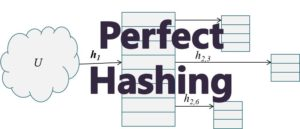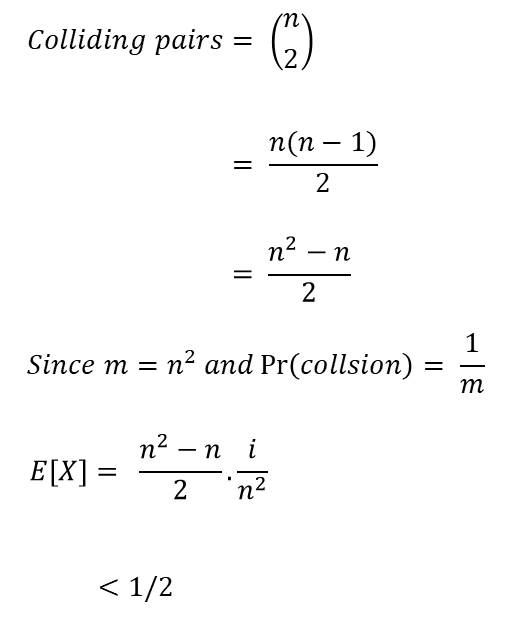# Perfect Hashing – How it Works

This is used when the keys stored in the hash table are expected to be static. In this case perfect hashing guarantees excellent average as well as worst-case performance. This is how real dictionaries work. You most of the time just need to read from a dictionary.Some application of perfect hashing includes:

• data storage on a CD ROM
• set of reserved words in a programming language

This hashing technique is called perfect hashing because it takes constant time, O(1) to search for an item in the table.

##### 1. Overview of Perfect Hashing

Perfect hashing is implemented using two hash tables, one at each level. Each of the table uses universal hashing. The first level is the same a hashing with chaining such that n elements is hashed into m slots in the hash table. This is done using a has function selected from a universal family of hash functions.

The second level of uses a second hash table (instead of a linked list used in chaining). Elements that hash to the same slot j in the first hash table are stored in a second hash table. This second hash table is known as secondary hash table . The hash function hj is carefully  chosen such that there are no collision in the secondary table.

To ensure there are no collisions in the secondary hash table  Sj,  we need to make the size mj of the secondary table equal to the square of the number of keys hashing into slot j in the first table. That is:

mj = nj2

The hash functions for the primary hash table is carefully chosen so that we limit the expected total amount of space used to be O(n). Take some time to watch the video explanation of Perfect Hashing.

##### 3. Choosing Hash Functions

The first level hash function is chosen from the universal hash family ℋ pm where p is a prime number larger than any key value. So the keys that hash into slot j of the first hash table are placed into a secondary hash table Sj using the hash function hj which is chosen from another family of hash functions ℋ p,mj. The size of the secondary hash table is mj.

The objective is to achieve a situation where there are no collisions in the secondary hash table. At least no expected collisions. Let’s examine the expected colliding elements.

##### 4. Estimating Expected Collisions

Theorem: Assuming n keys are stored in a hash table of size m = using a hash function h which is chosen randomly from a universal family of hash functions. Then the probability that there would be any collision is less than 1/2

Proof: There are 〈n choose 2〉 pairs of keys  that may collide. Each pair collides with a probability of 1/m if h is chosen randomly from a universal class ℋ  of hash functions. We use a random variable X to count the number of collisions.

Let E(X) = expected number of collisionsIt then follows that a hash function h that is chosen randomly from the set ℋ  is have a high likelihood of having no collisions.

##### 5. Analysis of Perfect Hashing

If  the number of keys n is small we can get away with using a hash table of size m = n2 and choosing a random has function from ℋ . But if n is large, the m = n2 would be expensive. Therefore, we resort to using two level hash hashing:

in the first level, hash function h is used to hash n keys into m slots where m = n. Then the number of keys hashing to slot j is nj.

in the second level, we now use a secondary hash table of size mj = nj2 which ensures a collision does not occur (given the table is static)

##### Check these Data Structure and Algorithms Tutorials#### kindsonthegenius

Kindson Munonye is currently completing his doctoral program in Software Engineering in Budapest University of Technology and Economics

View all posts by kindsonthegenius →

## 5 thoughts on “Perfect Hashing – How it Works”

1.Abraham Shawa says:

good lesson thank you so very much. though i would really appreciate if you could make it more simpler

1.kindsonthegenius says:

Thanks a lot.